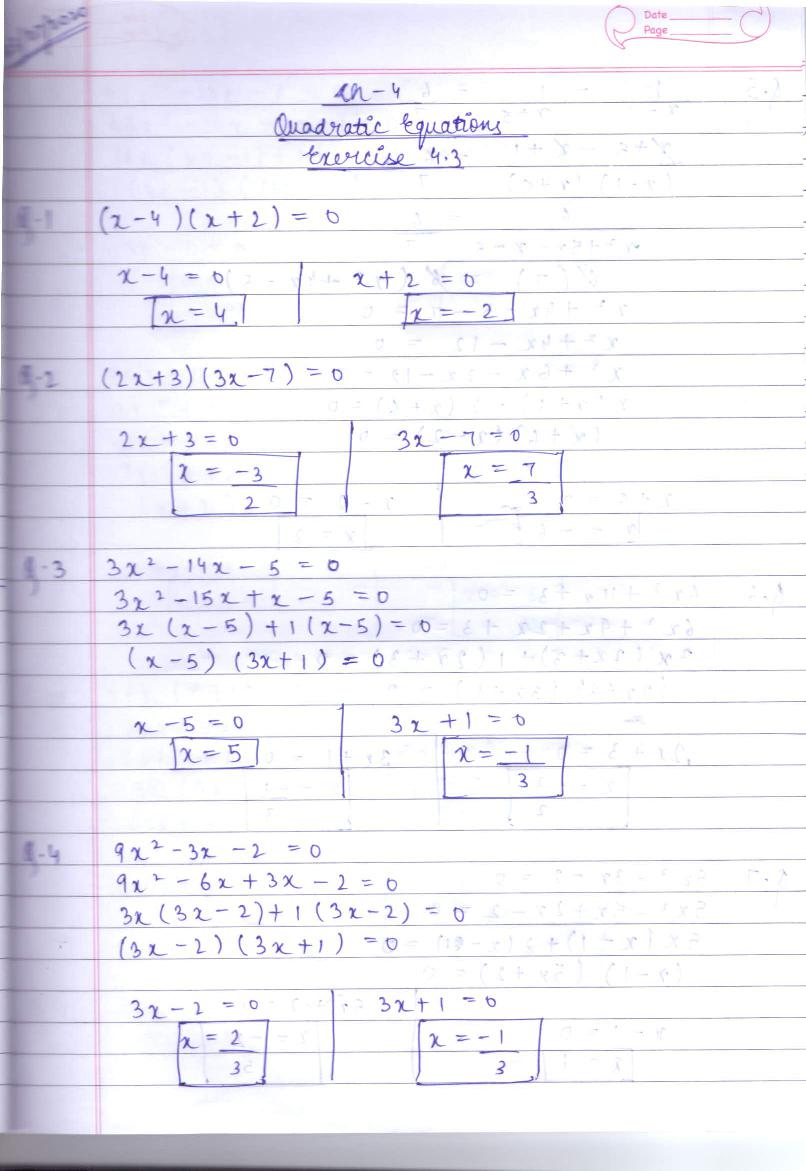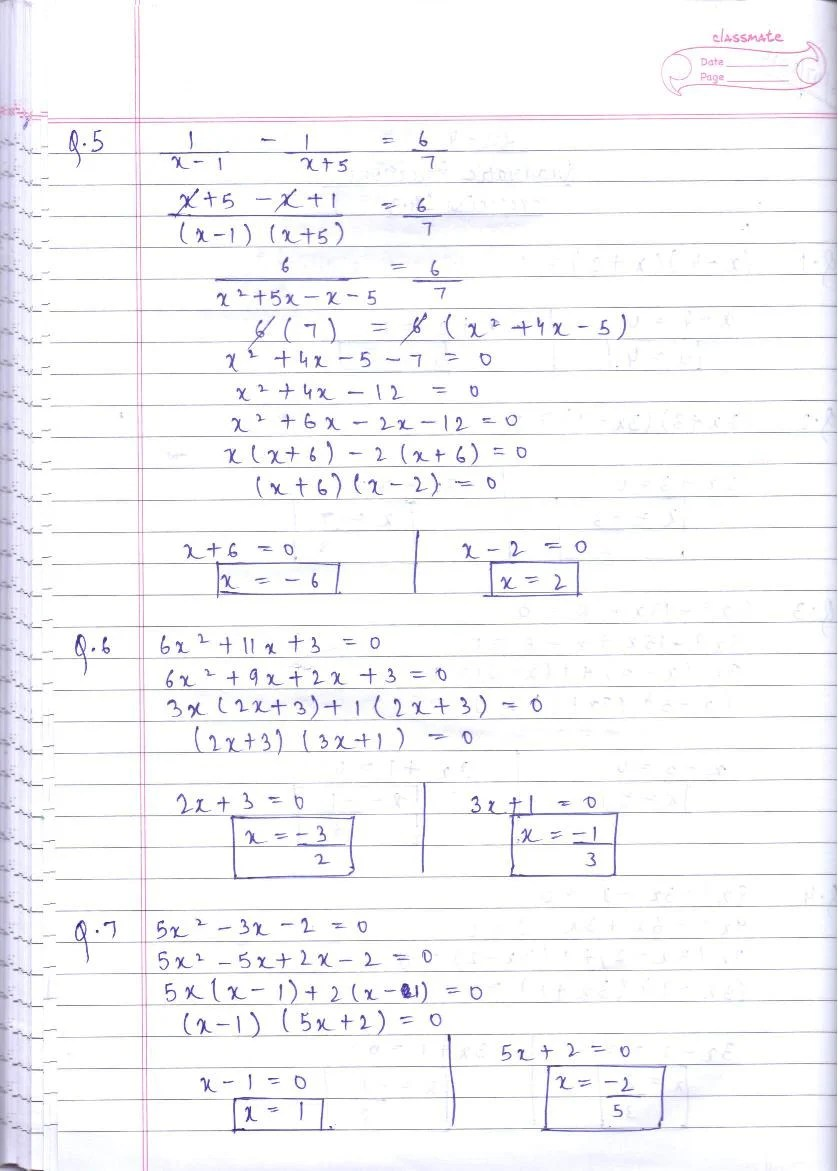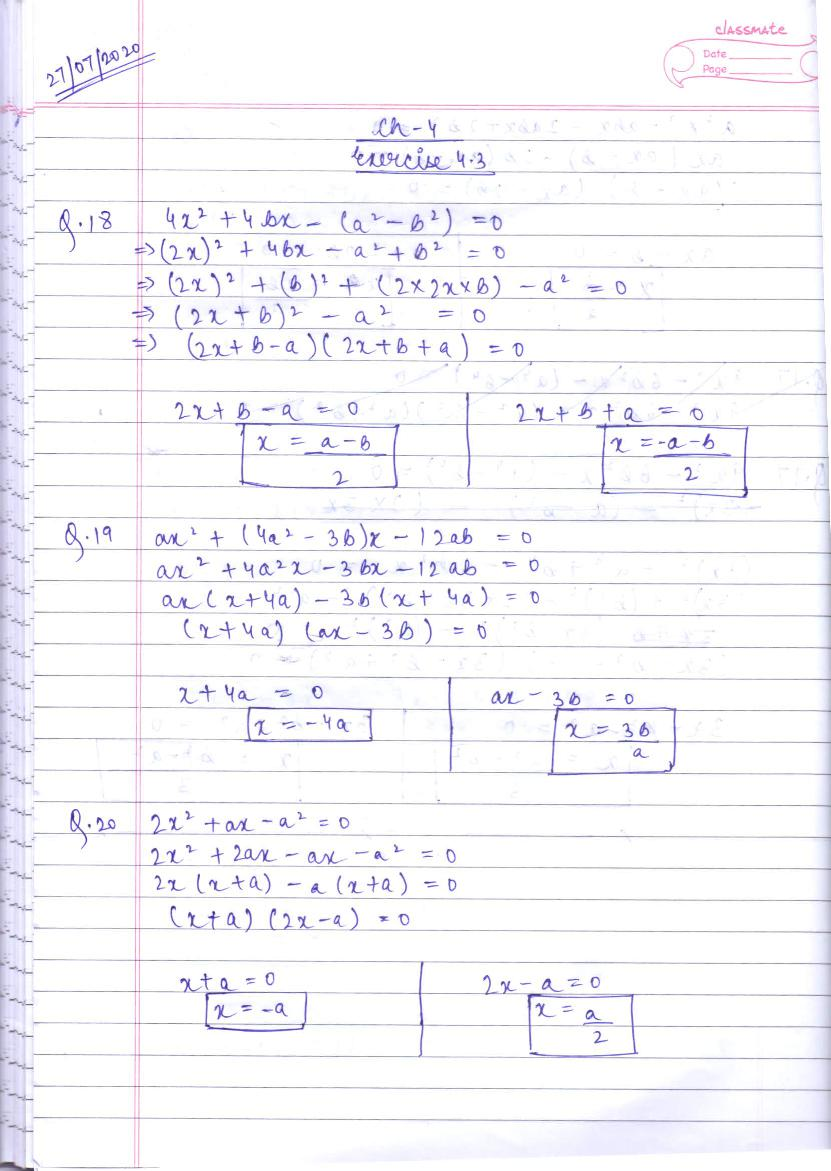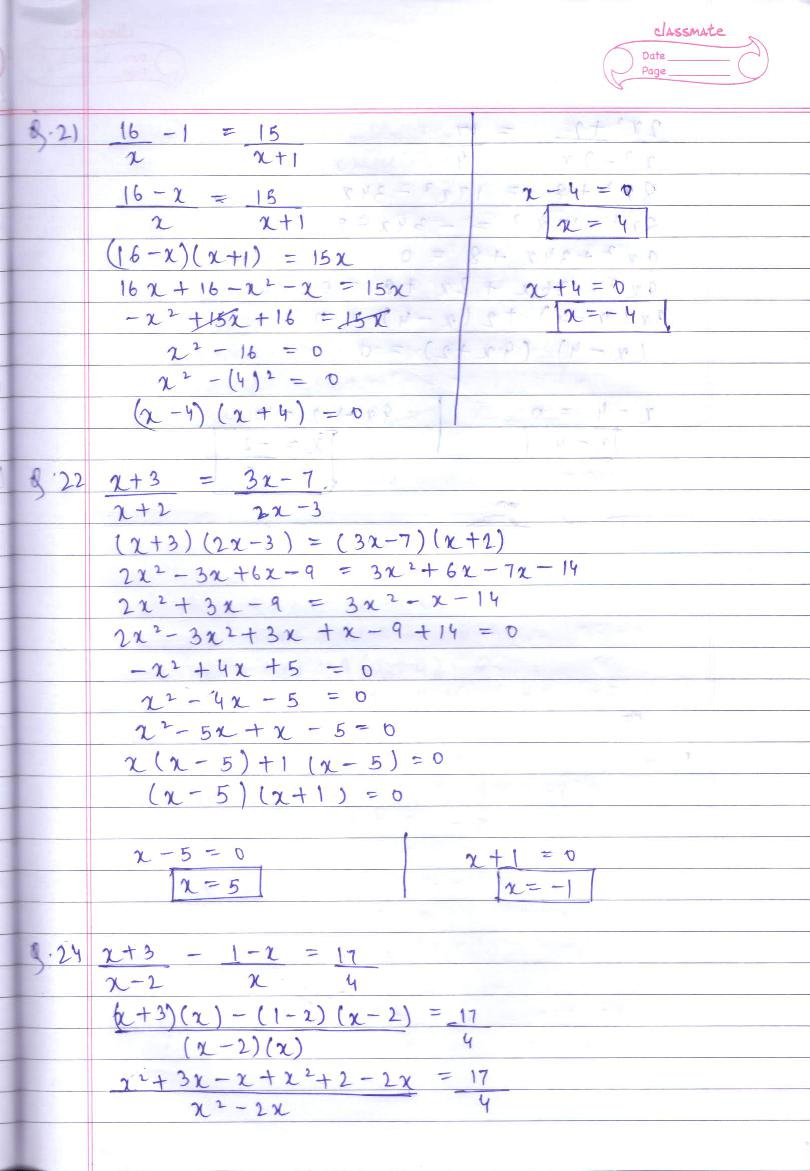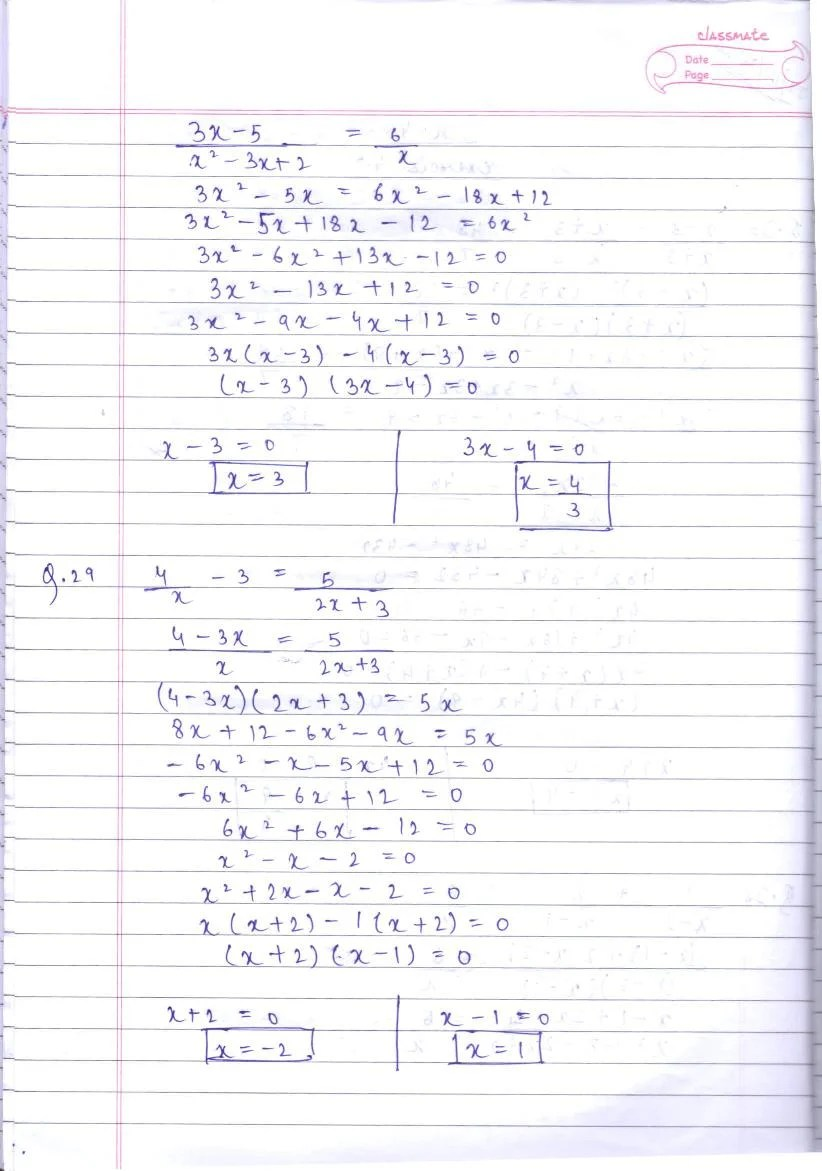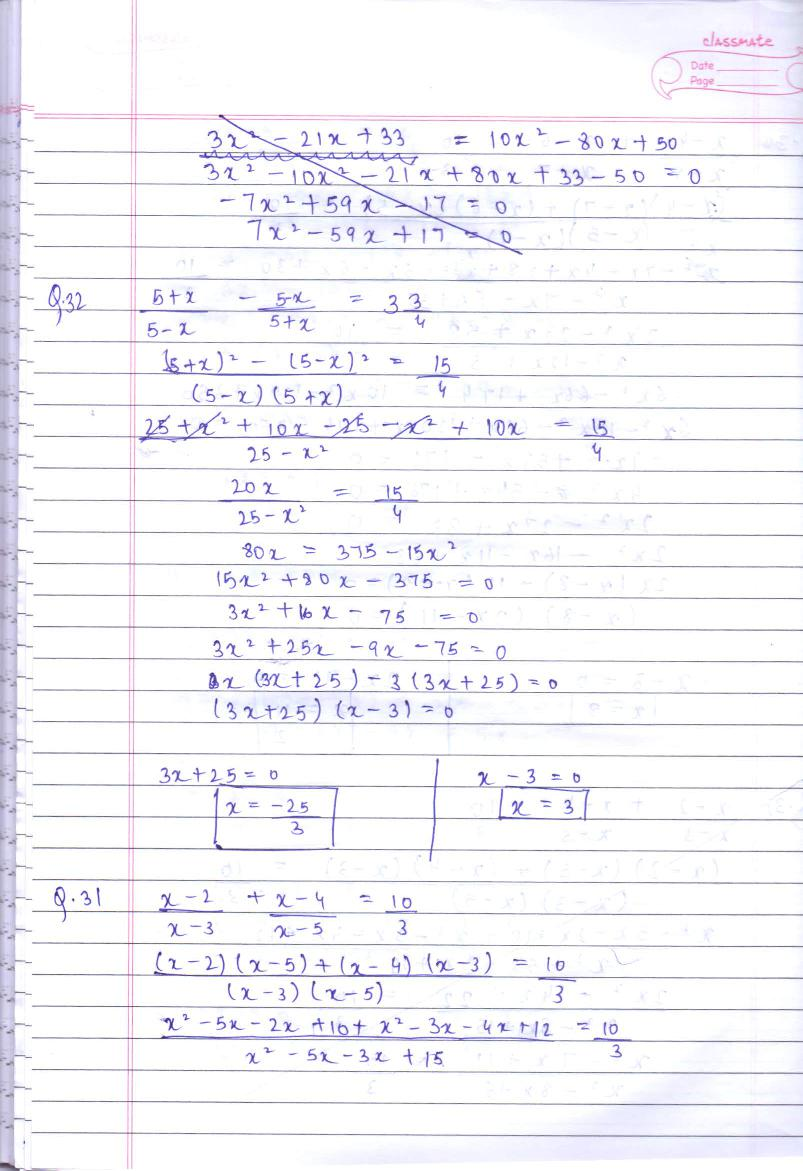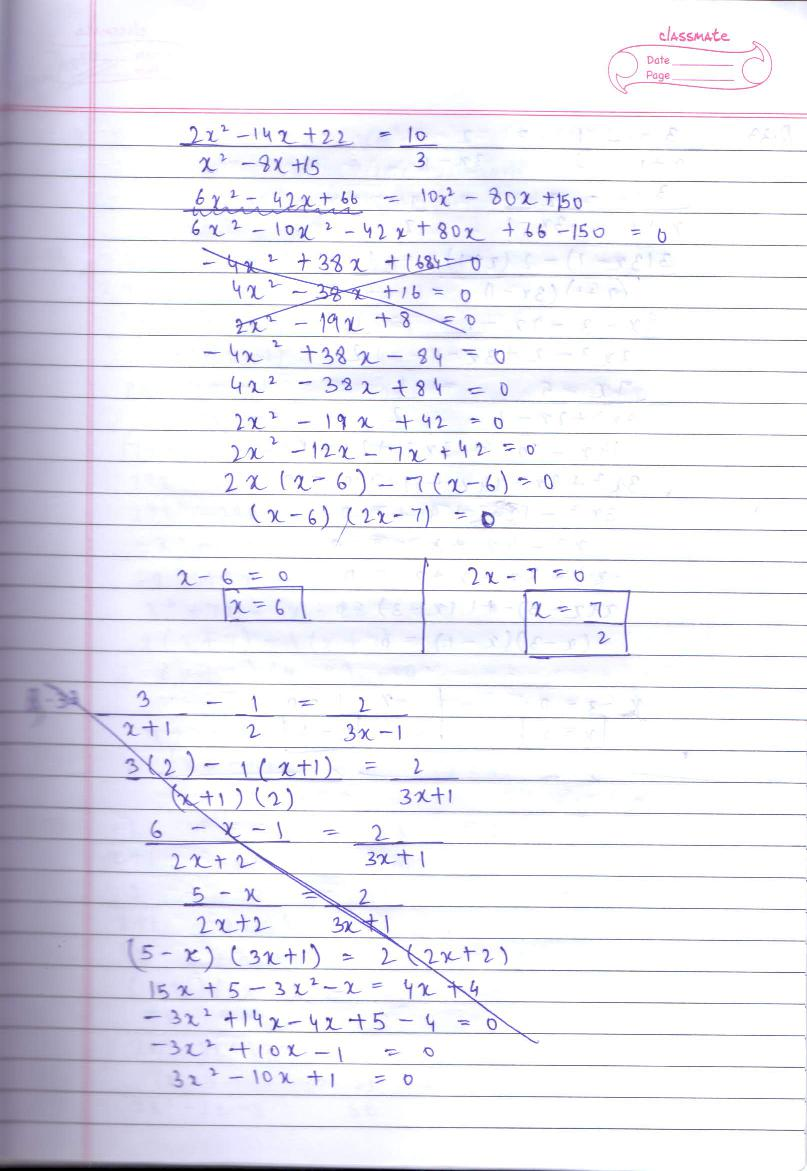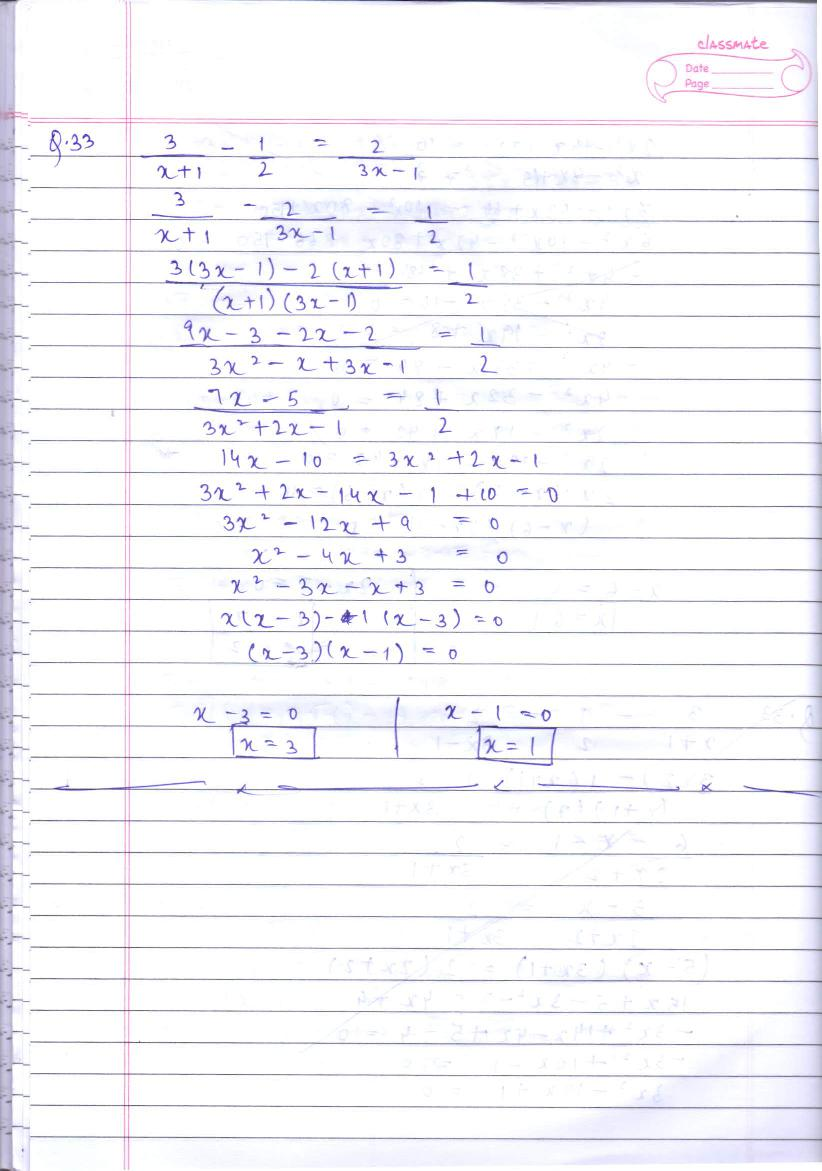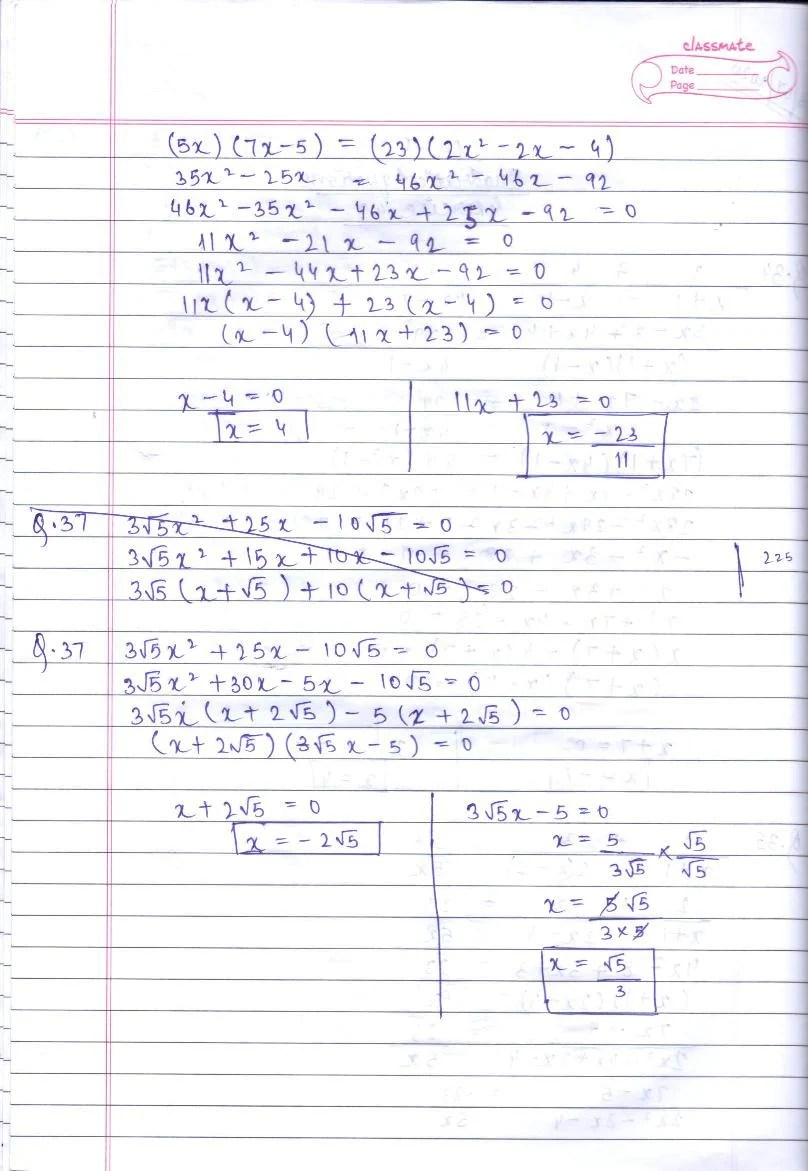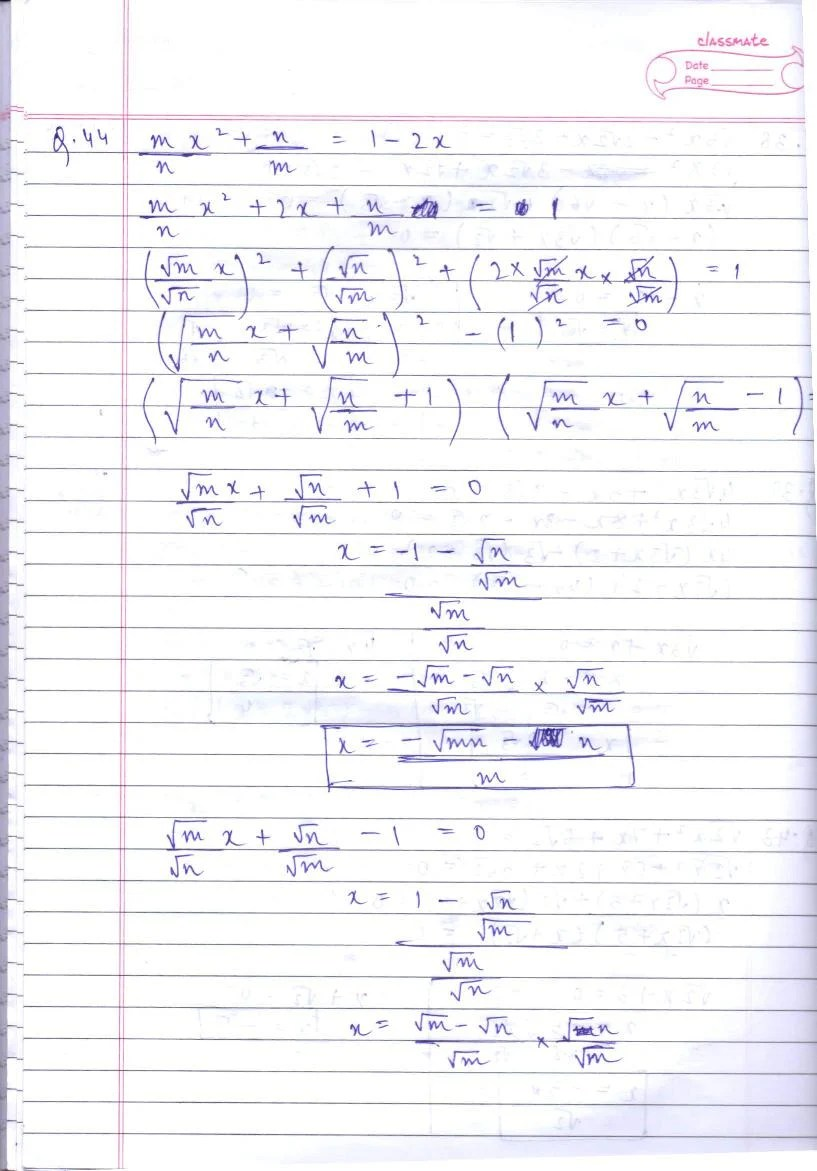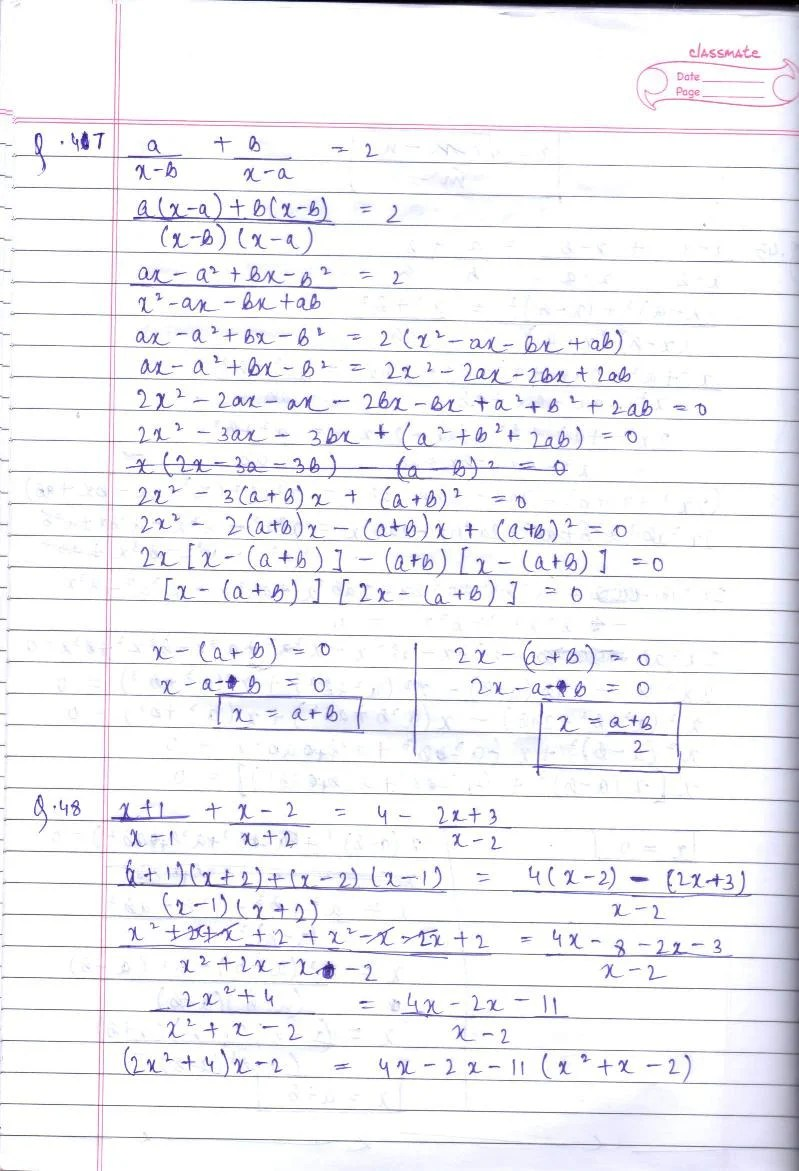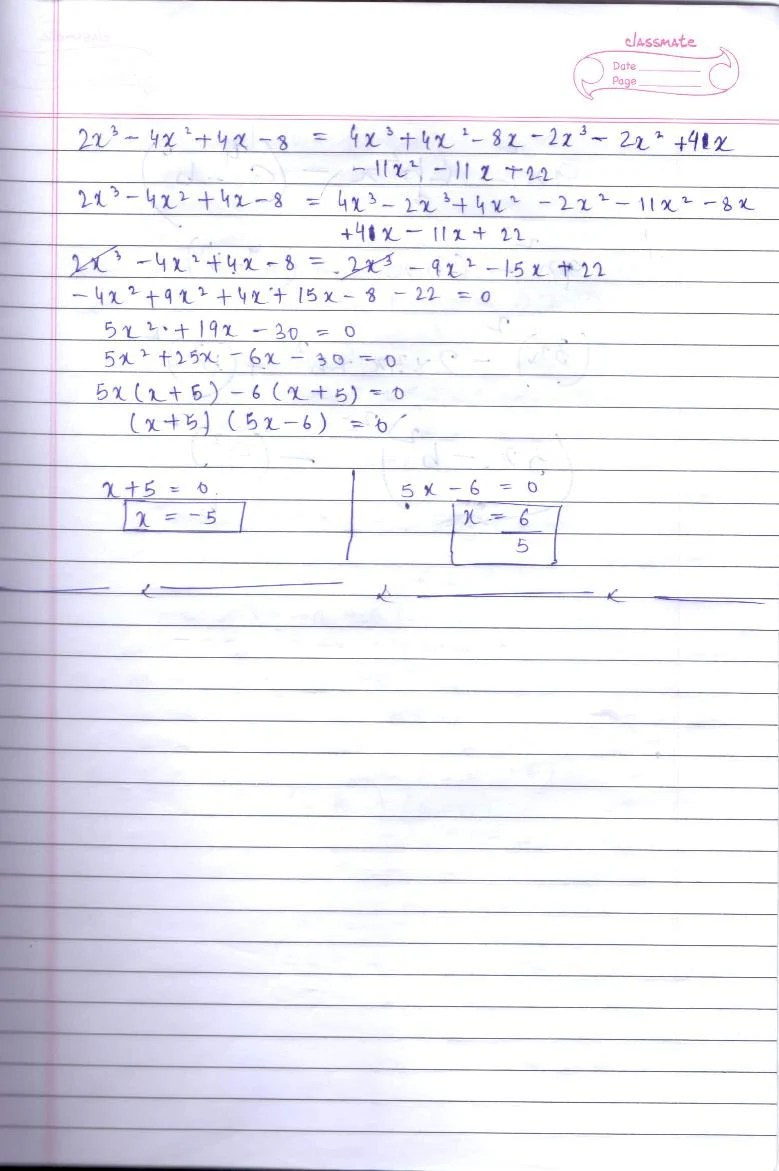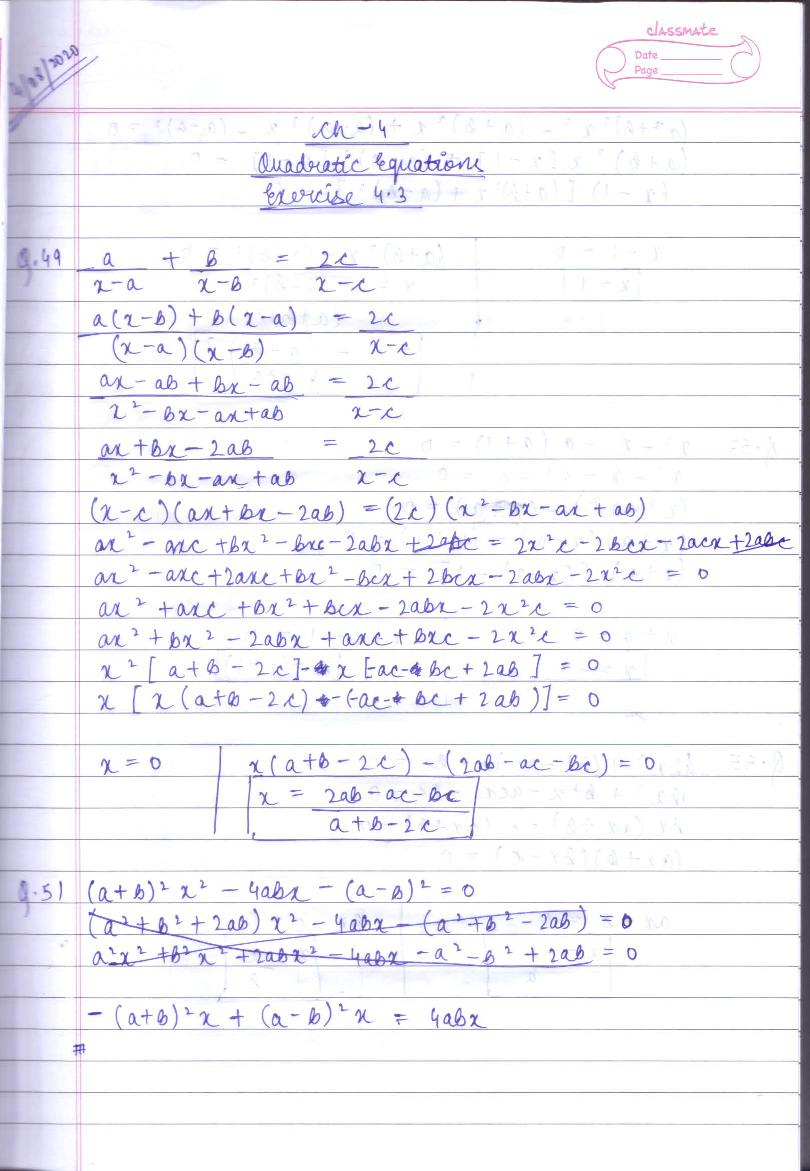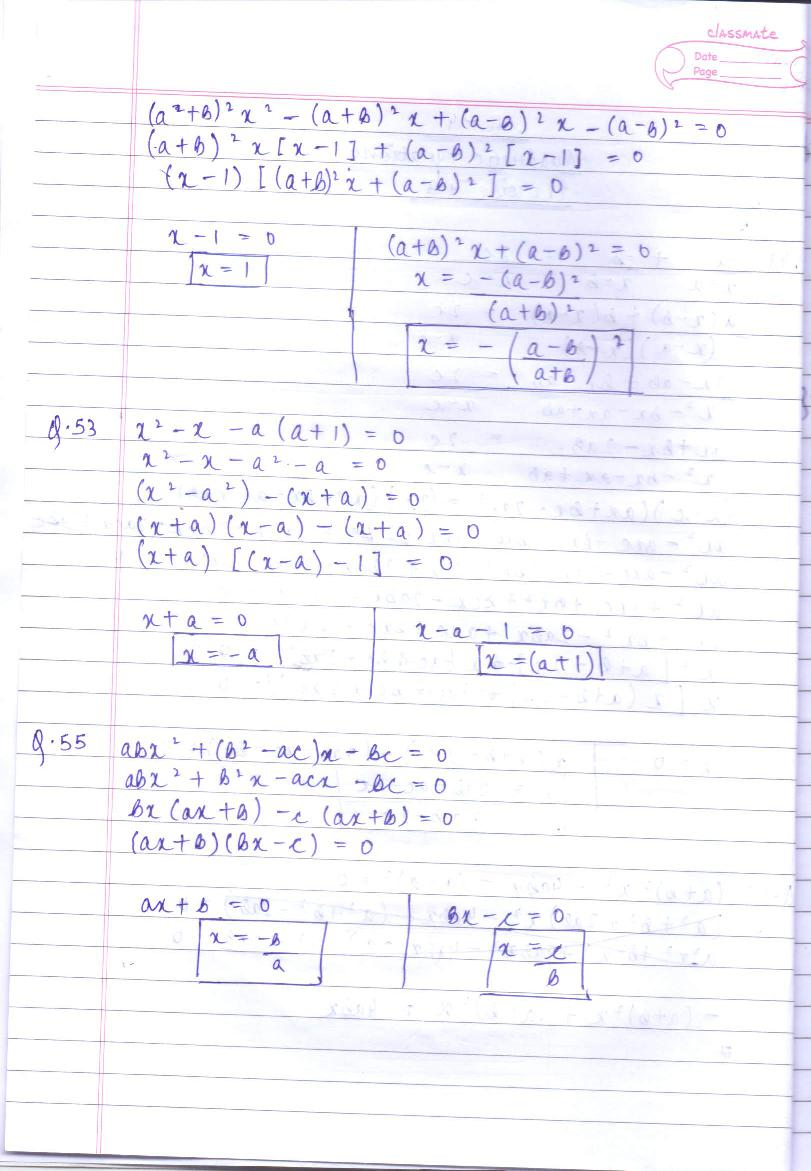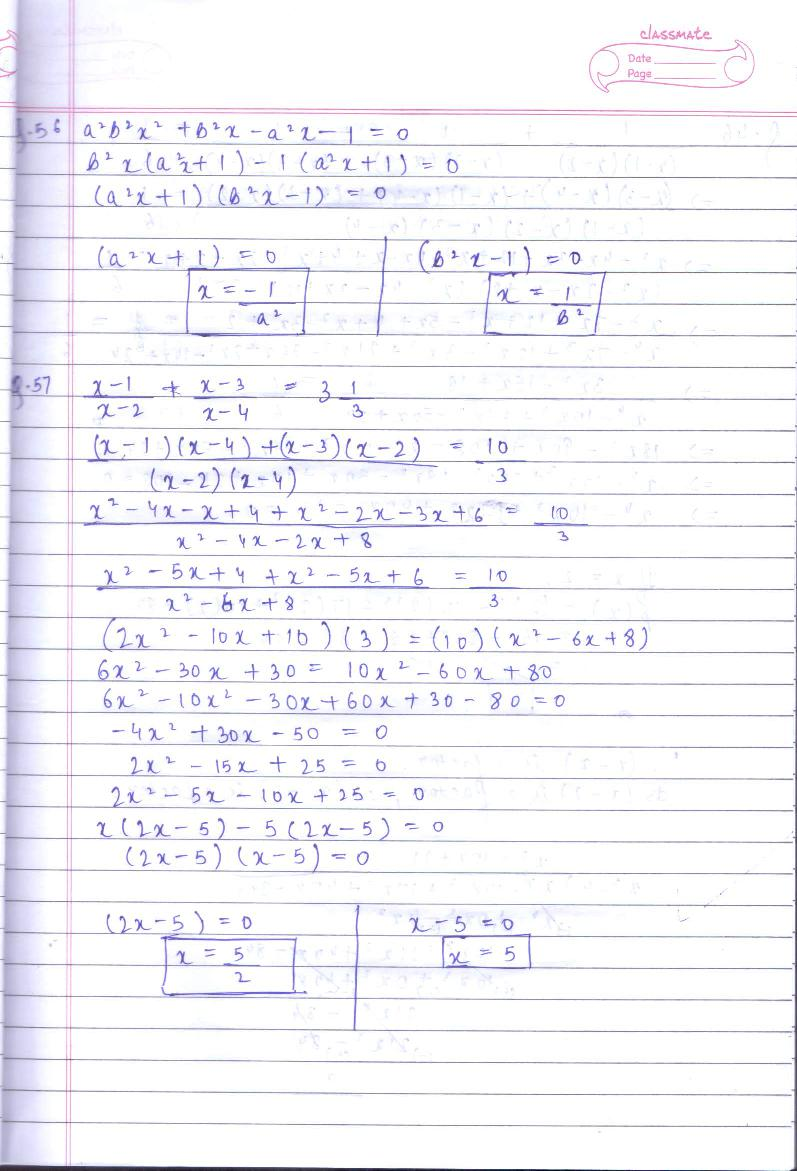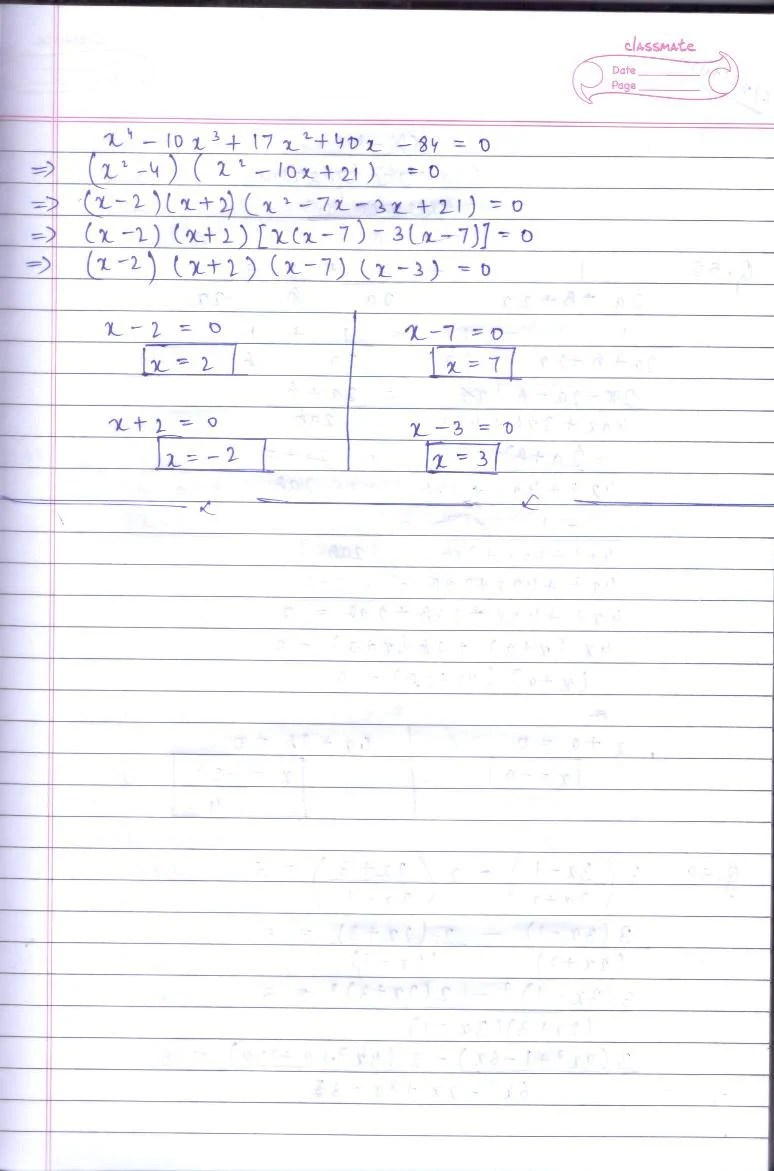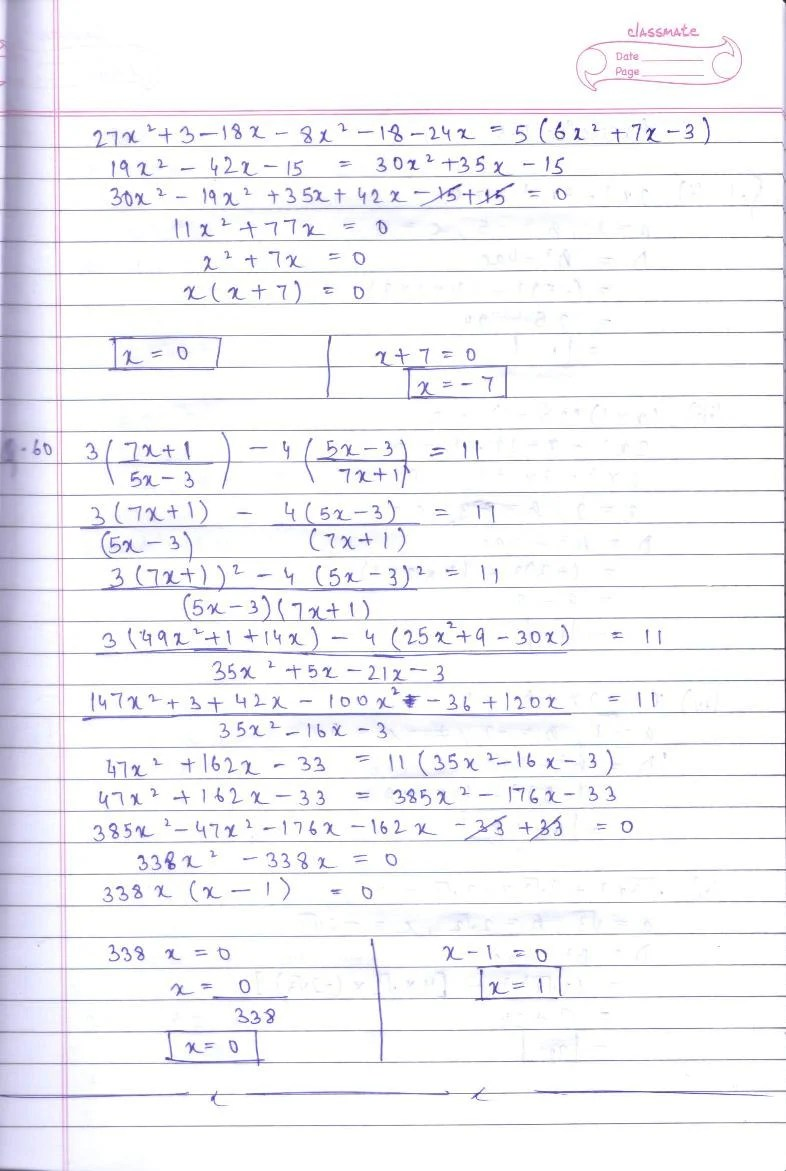# RD Sharma Solutions Class 10 Chapter 4 Quadratic Equations Exercise 4.4

Here you can get free RD Sharma Solutions for Class 10 Maths Chapter 4 Quadratic Equations Exercise 4.1. All RD Sharma Book Solutions are given here exercise wise for the chapter Quadratic Equations. RD Sharma Solutions are helpful in the preparation of several school level, graduate and undergraduate level competitive exams. Practicing questions from RD Sharma Mathematics Solutions for Class 10 Chapter 4 Quadratic Equations is proven to enhance your math skills.

 Class: Class 10th Chapter: Chapter 4 Exercise: Exercise 4.4 Name: Quadratic Equations

## RD Sharma Solutions Class 10 Chapter 4 Quadratic Equations Exercise 4.4

RD Sharma Class 10 Solutions Chapter 4 for Quadratic Equations Exercise 4.4 are given below.

RD Sharma Solutions Class 10 Chapter 4 Quadratic Equations Exercise 4.3